Here the details of the iguanodon series. Start with the Farey sequence $F(n)$of order n which is the sequence of completely reduced fractions between 0 and 1 which, when in lowest terms, have denominators less than or equal to n, arranged in order of increasing size. Here are the first eight Fareys

F(1) = {0⁄1, 1⁄1}
F(2) = {0⁄1, 1⁄2, 1⁄1}
F(3) = {0⁄1, 1⁄3, 1⁄2, 2⁄3, 1⁄1}
F(4) = {0⁄1, 1⁄4, 1⁄3, 1⁄2, 2⁄3, 3⁄4, 1⁄1}
F(5) = {0⁄1, 1⁄5, 1⁄4, 1⁄3, 2⁄5, 1⁄2, 3⁄5, 2⁄3, 3⁄4, 4⁄5, 1⁄1}
F(6) = {0⁄1, 1⁄6, 1⁄5, 1⁄4, 1⁄3, 2⁄5, 1⁄2, 3⁄5, 2⁄3, 3⁄4, 4⁄5, 5⁄6, 1⁄1}
F(7) = {0⁄1, 1⁄7, 1⁄6, 1⁄5, 1⁄4, 2⁄7, 1⁄3, 2⁄5, 3⁄7, 1⁄2, 4⁄7, 3⁄5, 2⁄3, 5⁄7, 3⁄4, 4⁄5, 5⁄6, 6⁄7, 1⁄1}
F(8) = {0⁄1, 1⁄8, 1⁄7, 1⁄6, 1⁄5, 1⁄4, 2⁄7, 1⁄3, 3⁄8, 2⁄5, 3⁄7, 1⁄2, 4⁄7, 3⁄5, 5⁄8, 2⁄3, 5⁄7, 3⁄4, 4⁄5, 5⁄6, 6⁄7, 7⁄8, 1⁄1}Farey sequences have plenty of mysterious properties. For example, in 1924 J. Franel and Edmund Landau proved that an asymptotic density result about Farey sequences is equivalent to the Riemann hypothesis.
More precisely, let a(n) be the number of terms in the Farey sequence F(n) (that is, a(1)=2,a(2)=3,…,a(8)=23 etc. This is sequence A005728 in the online integer sequences catalog).
Let $F(n)_j$ denote the j-th term in F(n), then the following conjecture is equivalent to the Riemann hypothesis

For every $\epsilon > 0$ there is a constant C depending on $\epsilon$ such that

$\sum_{j=1}^{a(n)} | F(n)_j – \frac{j}{a(n)} | < C n^{\frac{1}{2}+\epsilon}$

when n goes to infinity. Anyway, let us continue our construction. Farey sequences are clearly symmetric around 1/2 so let us just take half of them, so we jump to 1 when we have reached 1/2. Let us extend this halved Farey on both sides with $\infty$ and call it the modified Farey sequence f(n). For example,

$f(3) = {~\infty,0,\frac{1}{3},\frac{1}{2},1,\infty }$

Now consider the Farey code in which we identify the two sides connected to $\infty$ and mark two consecutive Farey numbers as

$$\xymatrix{f(n)_i \ar@{-}[r]_{\bullet} & f(n)_{i+1}}$$

That is, the Farey code associated to the modified sequence f(3) is

$$\xymatrix{\infty \ar@{-}[r]_{1} & 0 \ar@{-}[r]_{\bullet} & \frac{1}{3} \ar@{-}[r]_{\bullet} & \frac{1}{2} \ar@{-}[r]_{\bullet} & 1 \ar@{-}[r]_{1} & \infty}$$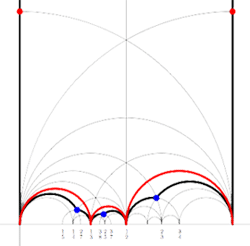Recall from earlier that to a Farey-code we can associate a special polygon by first taking the hyperbolic convex hull of all the terms in the sequence (the region bounded by the vertical lines and the bottom red circles in the picture on the left) and adding to it for each odd interval $$\xymatrix{f(n)_i \ar@{-}[r]_{\bullet} & f(n)_{i+1}}$$ the triangle just outside the convex hull consisting of two odd edges in the Dedekind tessellation (then we obtain the region bounded by the black geodesics for the sequence f(3)).

Next, we can associate to this special polygon a cuboid tree diagram by considering all even and odd vertices on the boundary (which are tinted red, respectively blue) together with all odd vertices in the interior of the special polygon. These are indicated in the left picture below. If we connect these vertices with the geodesics in the polygon we get a cuboid tree diagram. The obtained cuboid tree diagram is depicted on the right below.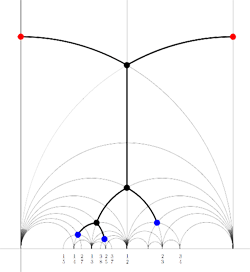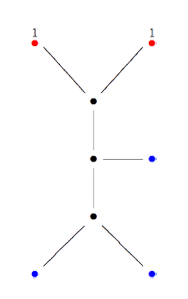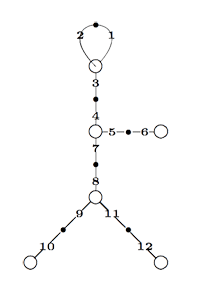Finally, identifying the red points (as they lie on geodesics connected to $\infty$ which are identified in the Farey code), adding even points on the remaining geodesics and numbering the obtained half-lines we obtain the dessin d’enfant given on the left hand side. To such a dessin we can associate its monodromy group which is a permutation group on the half-lines generated by an order two element indicating which half-lines make up a line and an order three element indicating which half-lines one encounters by walking counter-clockwise around a three-valent vertex. For the dessin on the left the group is therefore the subgroup of $S_{12}$ generated by the elements

$\alpha = (1,2)(3,4)(5,6)(7,8)(9,10)(11,12)$

$\beta = (1,2,3)(4,5,7)(8,9,11)$

and a verification with GAP tells us that this group is the sporadic Mathieu group $M_{12}$. This concludes the description of the second member of the Iguanodon series. If you like to check that the first 8 iguanodons are indeed the simple groups

$L_2(7), M_{12}, A_{16}, M_{24}, A_{28}, A_{40}, A_{48}, A_{60}, \ldots$

the following dissection of the Iguanodon may prove useful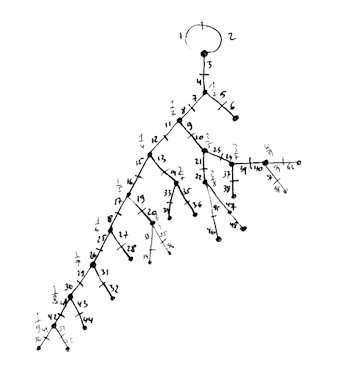Published in featured